# The WOOD1-World Instance by Wilson

To start first experiments we have to construct a concrete instance for the preceding gneral schema of a virtual world. We will gnerate such an instance following the ideas of Wilson (1974)  in his paper about most simple learning classifier systems.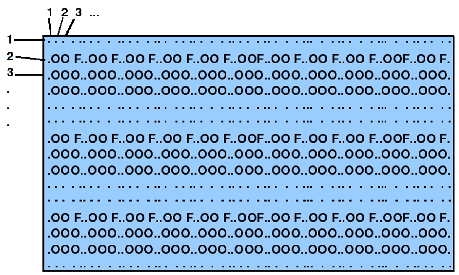In his paper describes Wilson a simple test environment called wood1. The overall layout (cf. figure 3.3) is a grid with 55 columns and 15 rows where a learning system called 'animat' will 'live' in this regular environment. The 'animat' is encded in the wood1-world as '*'.

We assume the following conventions (also with regard to the scilab software we are using):

1. The leftmost upper corner of the wood1 environment coincides with a 2-dimensional coordinate system starting with (1,1).
2. The X-axis runs from 'left' to 'right' in parallel with the columns of the matrix.
3. The Y-axis runs from 'up' to 'down' in parallel with the rows of the matrix.
4. Following Wilson we label the 'upper' row as north.

An animat can move in any of the eight directions as described in figure 3.4. Thus '00' encodes 'no move', '03' would encode a move to the right, etc. The environment is further the 'cause' for feedbacks also called rewards. For the consumption of food (F) Wilson proposes a value of '1000'. There are no further arguments in the paper of Wilson about the 'nature' of the rewards. Wilson assumes furthermore that the animat, if it plans to move onto a field which is occupied by an object (O), then the move is blocked. If the cell is occupied by food (F) then moving to the cell eats the food and gives the animat a feedback of an immediate reward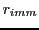.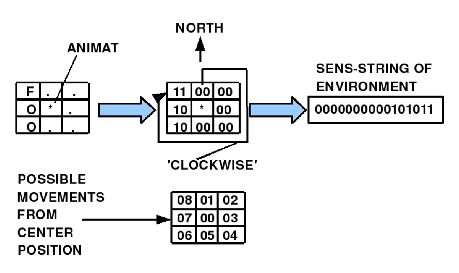One can think about possible reasons behind the amount of reward. To assume -as Wilson does in his paper- that the food is the only source of positive reward, this could be interpred as the consequence of the assumption that the ANIMAT as idealized biological system needs primarily energy. Food is some stuff which provides some amount of energy represented as 'amount of reward'. Additionally one can assume that the ANIMAT is consuming energy as long as it is not 'eating' some food. Thus energy consumption is a function of time given by the cycles. Doing nothing consumes energy and being active consumes even more energy. This leads to the working hypothesis, that

1. Doing no action consumes X1-many units of energy represented as negative reward. Proposal: '-1'
2. Doing an action as normal move consumes X2-many units of energy represented as negative reward. Proposal: '-2'
3. Doing an action as normal move with eating consumes X2-many units of energy and at the same time gains energy represented as positive reward. Proposal (Winston): '+1000'

Wilson assumes further some simple sensory perception of the ANIMAT including all the neighbouring cells starting with the cell 'in the north' and then going 'clockwise' around (cf. figure 3.4). If one wants to make systematic explorations of the dependency of the success from perception it would be convenient to have a more dynamic view of the perception model. We propose here two basic strategies of a scalable sensory perception (cf. figure 3.5):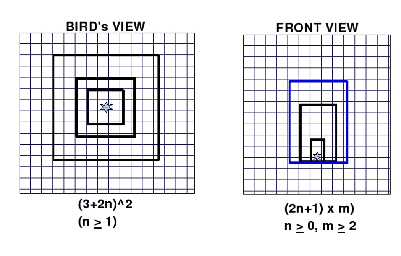Either the sensory perception will work like a bird's view having the ANIMAT in it's center and from this center it can be expanded outwards according to the formulawith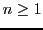or the sensory perception models a front view including the ANIMAT as part of this view with the formulawithand.

A bird's view would be 'translated' into a sensory string row by row using the encoding of Wilson. The same would happen in the case of a front view.

Assuming a front view induces some kind of a direction. Therefore we will 'keep this aside' as long as possible because this makes the world a lot more complicated.

Gerd Doeben-Henisch 2013-01-14